# 555 Timer – Pin Description & Applications

### IC 555 Pins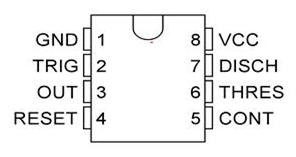##### Pin 1

It is the ground pin directly connected to the negative rail. It should not be connected using a resistor, because all the semiconductors inside the IC will heat up due to stray voltage accumulating in it.

##### Pin 2

It is the Trigger pin to activate the IC’s timing cycle. It is generally low signal pin and the timer is triggered when voltage on this pin is below one third of the supply voltage. The trigger pin is connected to the Inverting input of the comparator inside the IC and accepts negative signals. The current required for triggering is 0.5 uA for a period of 0.1uS.The triggering voltage maybe 1.67 V if the supply voltage is 5V and 5 V if the supply voltage is 15V. The triggering circuit inside the IC is too sensitive so that the IC will show false triggering due to noise in the surroundings. It requires a pull up connection to avoid false triggering.

##### Pin 3

It is the output pin. When the IC triggers via pin 2, the output pin goes high depending on the duration of the timing cycle. It can either sink or source current which is at maximum 200mA. For logic zero output, it is sinking current with voltage slightly greater than zero. For logic high output, it is sourcing current with the output voltage slightly lesser than Vcc.

##### Pin 4

It is the reset pin. It should be connected to the positive rail to work the IC properly. When this pin is grounded, the IC will stop working. The reset voltage required for this pin should be 0.7 volts at a current of 0.1mA.

##### Pin 5

Control pin – The 2/3 supply voltage point on the terminal voltage divider is brought to the control pin. It requires to be connected to an external DC signal to modify the timing cycle. When not in use, it should be connected to the ground through a 0.01uF capacitor; otherwise the IC will show erratic responses

##### Pin 6

It is the Threshold pin. The timing cycle is completed when voltage on this pin is equal to or greater than two-third of Vcc. It is connected to the non inverting input of the upper comparator so that it accepts the positive going pulse to complete the timing cycle. Typical threshold current is 0.1 mA as in the case of Reset pin. The time width of this pulse should be equal or greater than 0.1uS.

##### Pin 7

Discharge pin. It provides a discharge path for the timing capacitor through the collector of the NPN transistor, to which it is connected. The maximum allowable discharging current should be less than 50 mA otherwise the transistor may damage. It can be also used as an open collector output.

##### Pin 8

It is positive rail connected pin which is connected to positive terminal of the power supply. It is also known as Vcc.  IC555 works in a wide range of voltage from 5V to 18 V DC where as the CMOS version 7555 works with 3 Volts.

### Before getting into details about applications of 555 timer, let us have a brief about the 3 modes

Monostable mode

The output pulse width time t is the time taken to charge the capacitor to 2/3 of Vcc.

T=RC, where t in seconds, R in ohms and C in farads – 1.1 X RxC

Astable Mode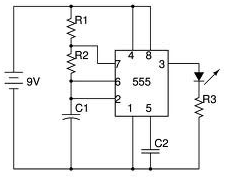T = t1+t2

t1 = 0.693(R1+R2)x C – Charging time

t2 = 0.693R2C – Discharging time

Frequency

f = 1 / T = 1.44 / (R1+2R2) C

Duty cycle

DC = (R1+R2) / (R1+2R2) X 100%

### 4 Applications of 555 Timers

#### 1. IR Obstructer using 555 Timer

From the below circuit, here we are using 555timer where pin1 is connected to ground (GND) and pin2 is connected to pin6 which is threshold pin of timer. The pin3 is connected to base of a transistor BC547 whose emitter is connected to GND and collector is connected to power supply through IR diode / LED D1 and a Resistor. The pin4 of timer is connected to pin7 through resistor R2 of 1k again pin7 and pin5 are shorted together in between two capacitors C1 of 0.01µF, C2 of 0.01µF and a potential divider of 2.2k. The pin8 of the timer is connected to power supply.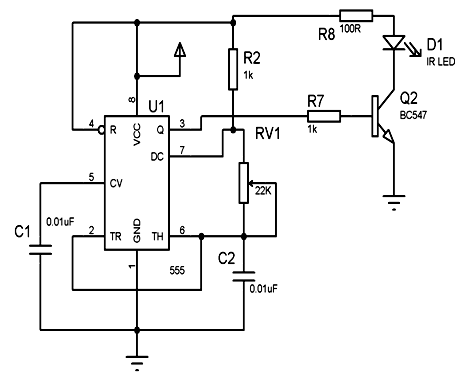In this, the 555 timer used is in free running astable multi-vibrator mode at a frequency of 38 KHz and a duty cycle of about 60%. The said pulses drives a transistor Q2 the collector of which powers an IR diode D1 through 100Ω resistor from the power supply 6V DC. As the receiving unit of any T.V receives 38KHz pulses from its own remote, continuous stream of 38KHz pulses so generated by an external timer circuit superimposes and overrides the remote signal resulting in making the T.V remote sent pulses scrambled. Thus the T.V is not able to respond the required pulses from the T.V remote to take any action such as channel change, volume up, down etc.

#### 2. IC 555 Tester: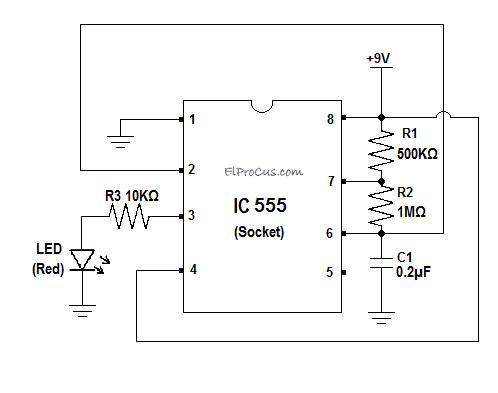The circuit is arranged as an astable multivibrator with R1 as 500 kilo ohm resistor (1/4 watt), R2 as 1 mega ohm resistor (1/4 watt) and C1 as 0.2 micro farad capacitor (ceramic bipolar). Connect this circuit with an empty 8 pin socket in place of the IC 555 so that you can easily attach the IC to be tested. Connect a power supply of 9v. You can use either a 9V adapter or else a 9V PP3 battery will work too. The resistors R1, R2 and C1 in the circuit above are used to set the frequency of operation of this circuit. As it is in astable mode, the output frequency of a 555 timer can be calculated by using the following formula:

The circuit operates at a frequency of 2.8Hz, i.e. the output turns ON and OFF approximately 3 times (2.8 Hz) every second. Pin-3 is the output pin of the 555 timer. We have connected an LED at the output pin in series with a 10KΩ resistor. This LED turns on when the pin-3 goes high. This means that the LED blinks with a frequency of approximately 3Hz.

I have soldered this circuit on a general purpose PCB for my personal use. Here is the hardware for it:You can see that the hardware can be made in just the size of a thumb and it does not cost much either. It is a very useful utility and saves a lot of time in testing 555 ICs. If you frequently work with 555 timers, I suggest you to have one with you. It really helps. It seems to be a simple circuit but its quite useful for all those who work with 555s.

#### 3. 60 Seconds Timer

Circuit Diagram: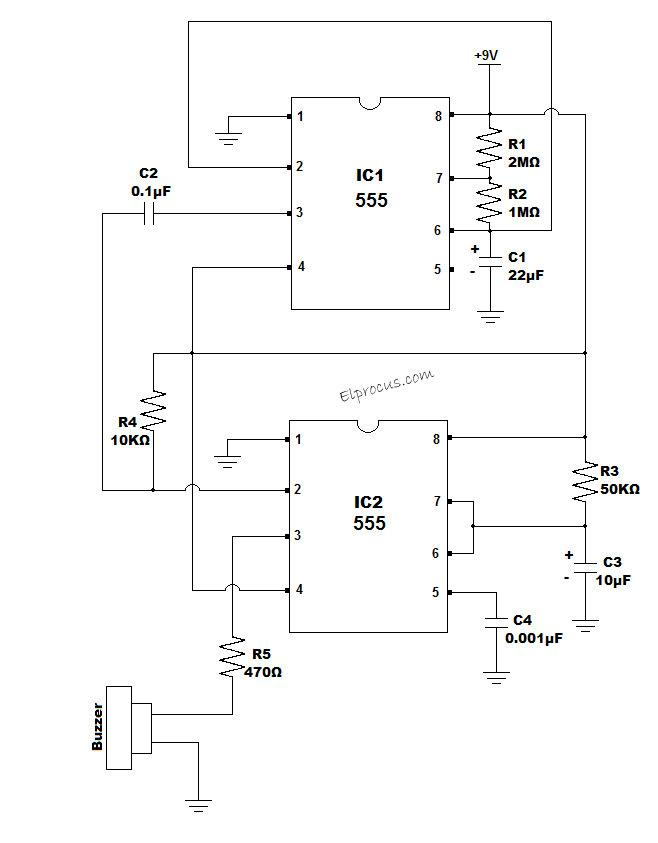### Circuit Operation:

Part-1 Astable:

The 555 timer IC1 in the above circuit is in the astable mode with R1=2MΩ, R2=1MΩ and C1=22µF. With this configuration, the circuit operates with a time period of approximately 60 seconds. We are now speaking in terms of time period instead of frequency because the frequency is too small so that mentioning it in time period will be convenient.

Here is the analysis of IC1:

The time period of the a stable multi vibrator depends on the values of resistors R1, R2 and capacitor C1. For the timer to have a time period of 60 seconds, tune the variable resistors R1 and R2 to the maximum range, i.e. R1=2MΩ and R2=1MΩ.

The time period is calculated by the formula:

T1=0.7(R1+2R2)C1

Here,

R1=2MΩ=2000000Ω

R2=1MΩ=1000000Ω

and C1=22µF

By substituting the above values in the above equation for time period, we get

T1=61.6 seconds

Considering the tolerance of the resistors and the capacitors, we can round off the value of time period to 60 seconds. When you are doing this project, I recommend you to check out the time period practically and adjust the values of the resistors accordingly so as to get an exact 60 seconds. I am telling you this because what all we do theoretically cannot be achieved exactly in practice.

Part-2 Mono stable:

Now we shall analyse the working of the 555 timer IC2. IC2 is connected in monostable mode. In monostable mode, the circuit will provide a HIGH output only for a defined time period T2 after it is triggered which is defined by the resistor R3 and the capacitor C3. The time period for T2 is given by the formula:

T2=1.1R3C3 (seconds)

Here,

R3=50KΩ,

and C3=10µF.

Substituting the values of R3 and C3 in the monostable time period equation we will get the time period as:

T2=0.55 seconds

This means that the output of IC2 (Pin3 of IC2) will remain HIGH for about 0.55 seconds when it is triggered and goes back to LOW state after that.

### How is the monostable circuit IC2 triggered?

The pin-2 of IC2 is the trigger input. It receives input from the pin-3 of IC1 which is the output pin of IC1. The capacitor C2 of 0.1µF transforms the square wave generated at the output IC1 into a positive and negative pulses so that the mono stable circuit IC2 can be negatively edge triggered. The triggering happens whenever the square wave at the output of the IC1 falls from HIGH voltage to LOW voltage.

The output of the mono stable circuit (IC2) stays HIGH until about half a second. In the time in which IC2 is HIGH, the output of IC2 (pin-3) drives the buzzer ON. This means the buzzer beeps for about half a second whenever IC2 is triggered. IC2 is triggered for every 60 seconds. This implies that the buzzer beeps at every 60 second interval.

Not just a 60 second timer. By adjusting the parameters of IC1, i.e. by varying the values of variable resistors R1 and R2, you can change the timing interval to your desired value. You can also change the value of C1 if required but it is not usually advisable as variable resistors are less costly and more rugged than variable capacitors.

#### 4. Cat and Dog Repellent Circuit

Normally audible frequency range which can be heard by human beings is about 20 KHz. However for many animals like dogs and cats, the audible frequency range can be as high as 100 KHz. This is basically because of the presence of erect ear flaps in dogs and cats compared to the lateral ear flaps of humans and the ability of dogs to move the ears in the direction of the sound. For dogs the high pitched noise emitted by household appliances like vacuum cleaners can be quite uncomfortable. Normally a dog hears less in the low frequency range and hears more in the high frequency range, in the ultrasonic range. This unique property of dogs makes them a relevant part of detection and survey teams where they can be used as hunting dogs by the police to hunt for missing persons or things.

This basic idea is utilized in this circuit to obtain a way to repel dogs from certain places. For example shunning away stray dogs from public places like malls, stations, bus stands etc.  The whole idea involves producing sound in the ultrasonic range so as to make the dogs uncomfortable and accordingly prevent them from approaching the areas.

The electronic dog repellent circuit diagram below is a high output ultrasonic transmitter which is primarily intended to act as a dog and cat repellent. The dog repellant uses a timer IC to give a 40 kHz square wave. This frequency is above the hearing threshold for humans but is known to be irritating frequency for dog and cats.

The system consists of a high power ultrasonic speaker which can produce sound in the ultrasonic range audible to the dogs. The speaker is driven by an H-bridge arrangement of 4 high power transistors, which are in turn driven by two timer ICs producing a 40 kHz square wave. The application of square waves can be scrutinized through a CRO. The output from the timers has low output current and hence the H-bridge arrangement is used to provide the necessary amplification. The H-bridge works by alternate conduction of the transistor pairs TR1-TR4 and TR2-TR3, which doubles the voltage across the ultrasonic speaker. The timer IC2 acts as a buffer amplifier which provides the H-bridge with an inverted input to that of the output of timer IC1.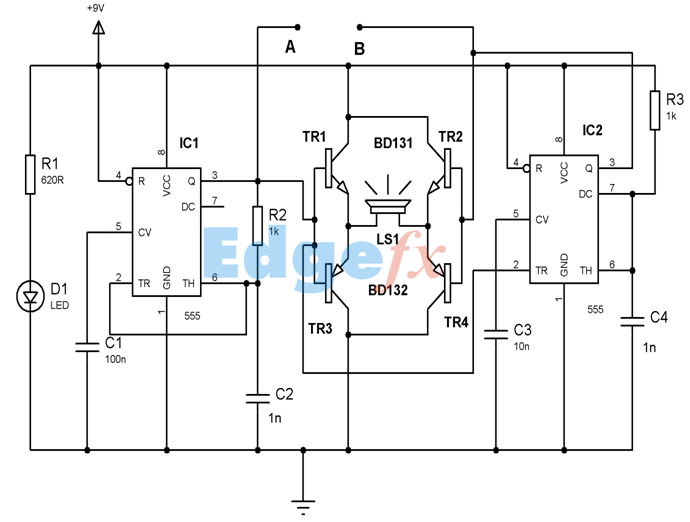An H-bridge network formed by 4 transistors is used as an amplifier, along with other timer IC and both timers being feeding inputs to H-bridge which can be seen at A & B in an oscilloscope.